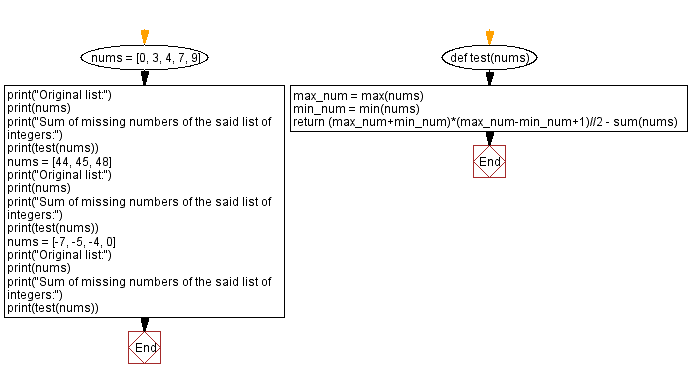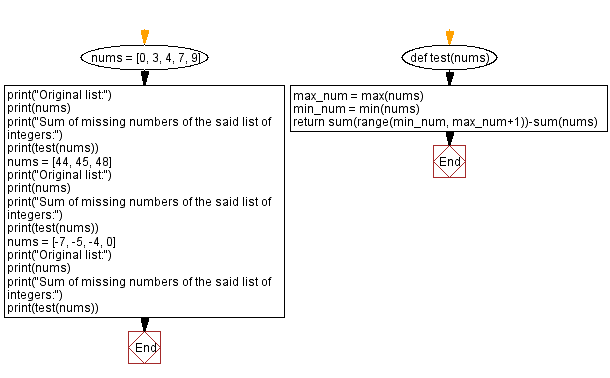﻿ Python - Sum of missing numbers of a list of integers# Python Exercises: Sum of missing numbers of a list of integers

## Python List: Exercise - 278 with Solution

Write a Python program to sum of missing numbers of a given list of integers.

Sample Data:
([0, 3, 4, 7, 9]) -> 22
([44, 45, 48]) -> 93
([-7, -5, -4, 0]) -> -12

Sample Solution-1:

Python Code:

``````def test(nums):
max_num = max(nums)
min_num = min(nums)
return (max_num+min_num)*(max_num-min_num+1)//2 - sum(nums)

nums = [0, 3, 4, 7, 9]
print("Original list:")
print(nums)
print("Sum of missing numbers of the said list of integers:")
print(test(nums))
nums = [44, 45, 48]
print("Original list:")
print(nums)
print("Sum of missing numbers of the said list of integers:")
print(test(nums))
nums = [-7, -5, -4, 0]
print("Original list:")
print(nums)
print("Sum of missing numbers of the said list of integers:")
print(test(nums))
```
```

Sample Output:

```Original list:
[0, 3, 4, 7, 9]
Sum of missing numbers of the said list of integers:
22
Original list:
[44, 45, 48]
Sum of missing numbers of the said list of integers:
93
Original list:
[-7, -5, -4, 0]
Sum of missing numbers of the said list of integers:
-12
```

Flowchart:## Visualize Python code execution:

The following tool visualize what the computer is doing step-by-step as it executes the said program:

Sample Solution-2:

Python Code:

``````def test(nums):
max_num = max(nums)
min_num = min(nums)
return sum(range(min_num, max_num+1))-sum(nums)

nums = [0, 3, 4, 7, 9]
print("Original list:")
print(nums)
print("Sum of missing numbers of the said list of integers:")
print(test(nums))
nums = [44, 45, 48]
print("Original list:")
print(nums)
print("Sum of missing numbers of the said list of integers:")
print(test(nums))
nums = [-7, -5, -4, 0]
print("Original list:")
print(nums)
print("Sum of missing numbers of the said list of integers:")
print(test(nums))
```
```

Sample Output:

```Original list:
[0, 3, 4, 7, 9]
Sum of missing numbers of the said list of integers:
22
Original list:
[44, 45, 48]
Sum of missing numbers of the said list of integers:
93
Original list:
[-7, -5, -4, 0]
Sum of missing numbers of the said list of integers:
-12
```

Flowchart:## Visualize Python code execution:

The following tool visualize what the computer is doing step-by-step as it executes the said program:

Python Code Editor:

Have another way to solve this solution? Contribute your code (and comments) through Disqus.

Previous Python Exercise: Largest, lowest gap between sorted values of a list.
Next Python Exercise: Check if each number is prime in a list of numbers.

What is the difficulty level of this exercise?

Test your Programming skills with w3resource's quiz.

﻿

## Python: Tips of the Day

Getting sorted iterable (can sort by "compare" function):

```>>> a = [1, 2, -3]
>>> sorted(a)
[-3, 1, 2]

>>> sorted(a,key=abs)
[1, 2, -3]
```